Courses

# Test: Torsion of Shafts - 3

## 10 Questions MCQ Test Topicwise Question Bank for Civil Engineering | Test: Torsion of Shafts - 3

Description
This mock test of Test: Torsion of Shafts - 3 for Civil Engineering (CE) helps you for every Civil Engineering (CE) entrance exam. This contains 10 Multiple Choice Questions for Civil Engineering (CE) Test: Torsion of Shafts - 3 (mcq) to study with solutions a complete question bank. The solved questions answers in this Test: Torsion of Shafts - 3 quiz give you a good mix of easy questions and tough questions. Civil Engineering (CE) students definitely take this Test: Torsion of Shafts - 3 exercise for a better result in the exam. You can find other Test: Torsion of Shafts - 3 extra questions, long questions & short questions for Civil Engineering (CE) on EduRev as well by searching above.
QUESTION: 1

Solution:
QUESTION: 2

### A shaft PQRS is subjected to torques at P,Q, R, S as shown in the given figure. The maximum torque for the shaft section occurs between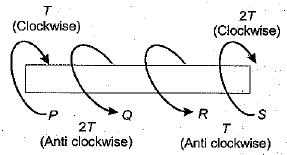Solution: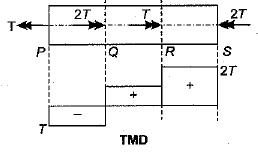QUESTION: 3

### A spring of stiffness 1 kN/m is stretched initially by 100 mm from the underformed position. The work required to stretch it by another 100 mm is

Solution:

k = 103/103 = 1N/mm
si = 100 mm
Fi = 1 x 100 = 100 N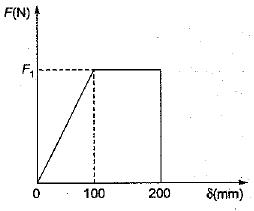Additional work required,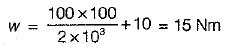QUESTION: 4

A close-coifed helical spring of stiffness 4 N/mm is in series with another spring of stiffness 6 N/mm. What is the stiffness of composite spring?

Solution:

Let the stiffness of composite spring = k
∴  1/k = 1/k1 + 1/k2
∴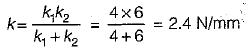QUESTION: 5

Stiffness of a close-coiled helical spring of wire diameter d, modulus of rigidity G number of coils n, mean coil radius R, is

Solution:
QUESTION: 6

Wahl’s factor takes into account

Solution:
QUESTION: 7

A spring with 25, active Coils cannot be accommodated within a given space. Hence 5 coils of the spring are cut. What is the stiffness of the new spring.

Solution:

As we know, k ∝ 1/n
∴ k2/k1 = n1/n2 = 25/20 = 1.25
⇒ k2 = 1.25 k1

QUESTION: 8

A close-coiled helical spring has 100 mm mean diameter and is made of 20 turns of 10 mm diameter steel wire. The spring carries an axial load of 100 N. Modulus of rigidity is 84 GPa. The shearing stress developed in the spring in N/mm2 is

Solution:

Closed coiled helical spring subjected to axial load (W) means that every section is subjected to torsion of WR where R is the radius of spring. From torsion formula,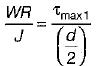d= diameter of wire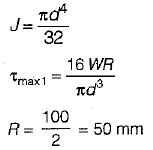d = 10mm
W = 100 N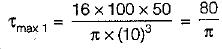Shear stress due to load,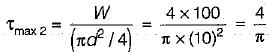QUESTION: 9

A carriage spring of span 'l’ consists of n plates each of thickness ‘t' and width b. If the spring carries a load w and modulus of elasticity of the spring is E, then the central deflection of the spring is

Solution:
QUESTION: 10

A solid shaft of diameter d is subjected to twisting moment T, such that the maximum shear stress developed is τ. Now a hole of 0.5 d diameter is drilled throdghout the length of the shaft along the axis. How much torque Tmust be reduced so that shear stress remain the same?

Solution: